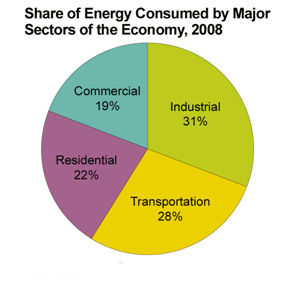FAQ
Home » Blog » FAQ » Can I operate a 50Hz transformer at 60Hz power supply?

# Can I operate a 50Hz transformer at 60Hz power supply?

Well first let get one thing straight for transformers: the higher the line frequency, the lower the core (iron) losses! The core power loss are proportional to kf*B^2 approximately for any machine, dynamic or static. But transformers are self-excited static machines, meaning the flux density B is reverse proportional to the line frequency, therefore Pcoreloss = kB^2*f=k*(1/f)^2*f=k/f... so the higher f, the lower the losses. However, increasing the frequency also increases the magnetizing inductance - lowering the magnetizing current. For if you increase the frequency you may want to increase the voltage. But of course this is not usually practical, as line voltage of 60Hz systems is usually lower than those of 50Hz systems. So operating a 50Hz motor at 60Hz should be safe, but may result in higher voltage drop because of lower magnetizing current and because of higher leakage inductance (the series inductance).

It is true that the higher the frequency, the higher the hysteresis (and eddy current) losses will be. But is it a common misconception to assume higher power losses when frequency increases in a transformer. Simply because the hysteresis losses depends not only on frequency, but on the max magnetic flux density as well (Bmax^2). The flux density is reversely proportional to the line frequency, which eventually causes lower core losses as you raise the frequency. This holds true for low and mid frequency ranges. For higher frequencies, skin effect and eddy currents dominates, so the picture may be different. However, iron core transformers do not operate in such high frequencies. We use ferrite core instead. In a practical transformer model, the core losses are represented by a parallel resistor (Rc). The resistor's value is linearly dependent of the line frequency (Rc=k*f), and the core losses are given by Pc=U^2/Rc... Of course this model is limited to mid-low frequencies...

Calculate (7 + 8) =

You may also like:

The rotating electrical machines have basically three noise sources: The ventilation system The rolling bearings Electromagnetic excitation Bearings in perfect conditions ...
The voltage transient which occurs whenever there is a sudden change in current in an inductive device. Inductors resist a sudden current change. V=L di/dt In electric motors this occurs at start up when the ...
I have a generator of 3 hp, and it outputs 230 V, and I have a submersible Electric Pump, the motor of which is rated to operate at 460 V, Can I use a step up transformer to increase the voltage output from my ...
When a choosing a power cable for a motor, we prefer using one larger diameter cable than two smaller diameter cables in parallel, although it would cost less to do so. Why?
Making a connection of 3 phase motor the nameplate shows different voltages for delta it is 380-400 volt and 660-690 volt for star, what option should be selected? the supply Line to Line voltage is ...Gozuk Blog: all about electric motor control & drives industries development in energy saving applications.

Featured

Like pumps, fans consume significant electrical energy while serving several applications. In many plants, the VFDs (variable ...A frequency inverter controls AC motor speed. The frequency inverter converts the fixed supply frequency (60 Hz) to a ... Motor starter (also known as soft starter, motor soft starter) is a electronic device integrates soft start, soft stop, ... Soft starter allows the output voltage decreases gradually to achieve soft stop, in order to protect the equipment. Such as the ... Soft Starter reduces electric motor starting current to 2-4 times during motor start up, reduces the impact to power grid during ...

In Discussion